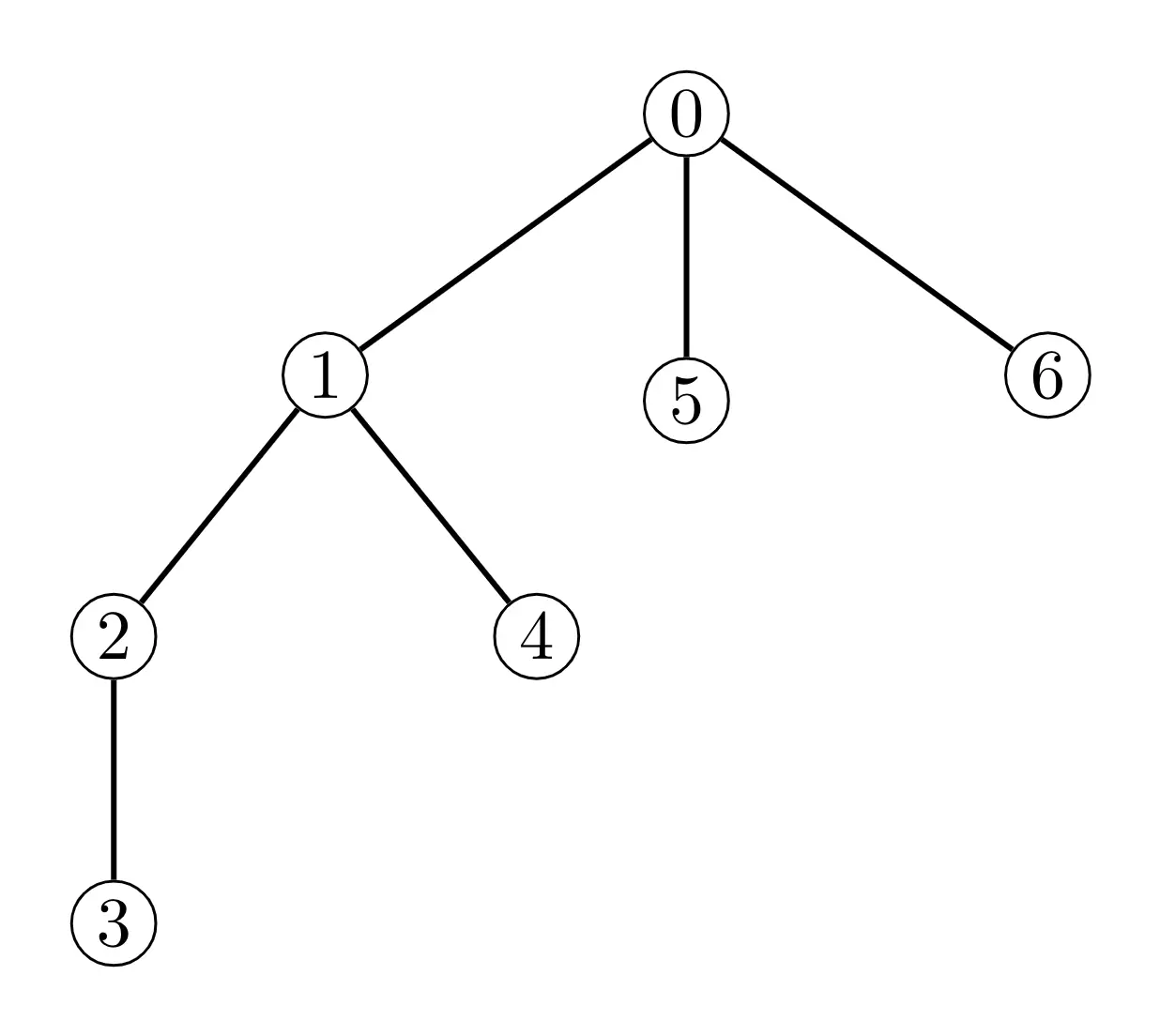# #557. 【APIO2020】有趣的旅途

### 实现细节

std::vector<int> createFunTour(int N, int Q)

• $N$: 一个整数表示景点的数量。

• $Q$: 一个整数表示询问次数的最大值。

int hoursRequired(int X, int Y)
• $X$: 一个整数表示第一个景点的编号。
• $Y$: 一个整数表示第二个景点的编号。
• 该函数将返回一个整数表示从第 $X$ 个景点到第 $Y$ 个景点需要花费的小时数。
• 如果 $X$ 或 $Y$ 的值不在 $0$ 到 $N - 1$ 的范围内，该测试点将视为答案错误。
int attractionsBehind(int X, int Y)
• $X$: 一个整数表示第一个景点的编号。
• $Y$: 一个整数表示第二个景点的编号。
• 该函数将返回一个整数表示有多少个景点 $Z$ 满足，当你想从第 $X$ 个景点到达第 $Z$ 个景点时一定会经过第 $Y$ 个景点。
• 如果 $X$ 或 $Y$ 的值不在 $0$ 到 $N - 1$ 的范围内，该测试点将视为答案错误。
• 该函数必须返回一个长为 $N$ 的整数序列，表示你找到的景点参观顺序。

### 样例评测库

N Q
A B
A B
.
.
.
A[N-2] B[N-2]

### 样例一

#### input

7 400000
0 1
0 5
0 6
1 2
1 4
2 3



#### output

3 6 4 5 2 0 1



#### explanation### 限制与约定

• $2 \le N \le 10^5$
• $Q = 4\times 10^5$
• 任意两个景点间可以通过双向道路互相到达。
• 每个景点至多连接着三条道路。

#### 子任务 $1$（$10$ 分）

• $N \le 17$

#### 子任务 $2$（$16$ 分）

• $N \le 500$

#### 子任务 $3$（$21$ 分）

• 对所有的 $1 \le i \lt N$，有一条连接着第 $i$ 个景点与第 $\left\lfloor\frac{i - 1}{2} \right\rfloor$ 个景点的双向道路。

#### 子任务 $4$（$19$ 分）

• 存在至少一个景点 $T$ 使得对于所有 $0 \le i \lt N$，hoursRequired(T, i) $\lt 30$ 并且存在一个整数区间 $[L_i, R_i](0 \le L_i \le i \le R_i \lt N)$ 满足下列条件：

• 从第 $T$ 个景点到达第 $j$ 个景点必须经过第 $i$ 个景点当且仅当 $L_i \le j \le R_i$。
• 若 $L_i \lt i$，则恰有一个景点 $X$ 满足：
• $L[i] \le X \lt i$
• 有一条连接第 $i$ 个景点与第 $X$ 个景点的道路。
• 若 $i \lt R_i$，则恰有一个景点 $Y$ 满足：
• $i \lt Y \le R_i$
• 有一条连接第 $i$ 个景点与第 $Y$ 个景点的道路。

#### 子任务 $5$（$34$ 分）

• 无附加限制。

1 1, 2, 3, 4
2 1, 2, 3, 4, 5, 6, 7
3 1, 2, 5, 8
4 1, 3, 6, 9
5 1, 2, 3, 4, 5, 6, 7, 8, 9, 10